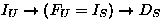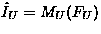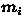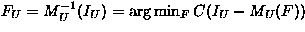Next: Measuring the description length Up: Introduction Previous: Introduction

## MDL-based cost functions for supervised and unsupervised learning

A common problem in pattern recognition is that one wants to recognise objects in a given raw data. One might have a large amount of raw data available, but only a small amount of labelled data. The typical approach is to extract features from the raw data in an unsupervised manner and then to find a mapping from the features to the corresponding labels (desired outputs) in a supervised manner. The whole process can be described by, where the arrows stands for mappings, I for input, F for feature, D for desired output, U for unsupervised and S for supervised.

Let us denote the model by M. In supervised learning one wants to find a mapping from the input data IS to the desired output data DS. The mapping is described by the model MS, whose structure and parameters are to be estimated. In a conventional neural network, the cost function C measures the error in the mapping: C(DS - MS(IS)). The MDL-based cost function for supervised learning is L(DS | MS, IS) + L(MS): the description length of the output data given the model and the input data plus the description length of the model. The first term corresponds to C(DS - MS(IS)) and the second term penalises for the complexity of the model. The input is assumed to be known by both the sender and the receiver and is not included in the description length.

In unsupervised learning one wants to find features FU which compactly describe the input data IU. The quality of the features is evaluated by estimating how much information about inputs they preserve, that is, how well the inputs can be reconstructed from the features. We shall consider a generative model MU which defines the reconstruction mapping:. The reconstruction error which measures the quality of the features is then C(IU - MU(FU)). We shall use vector quantisation to illuminate the use of a generative model.

In vector quantisation, the model consists of model vectors, which directly define the reconstruction mapping MU: given an index i, the reconstruction is. The index i thus plays the role of the feature. The quality of the feature is measured with the reconstruction error C, or quantisation error as it is often called in connection with vector quantisation. The feature is defined as the index that minimises the reconstruction error, or to put it in other words, the index of the closest model vector:.The model can be adapted by minimising the reconstruction error, that is, by moving the closest model vector even closer to the input. The features and the model are thus both defined as the minimum of the reconstruction error.

In addition to the term measuring the reconstruction error, the MDL-based cost function for unsupervised learning has terms penalising the complexity of the model and the features: L(IU | MU, FU) + L(MU) + L(FU). The cost function is used in exactly the same way as with vector quantisation: the features and the model are found by minimising it.

The features are often considered a subset of the parameters of the model, because they too have to be estimated from the data and are included in the cost function. The only difference between the features and the parameters is that the features change for each input element, whereas the parameters are constant for different data elements.Next: Measuring the description length Up: Introduction Previous: Introduction
Harri Lappalainen
5/19/1998